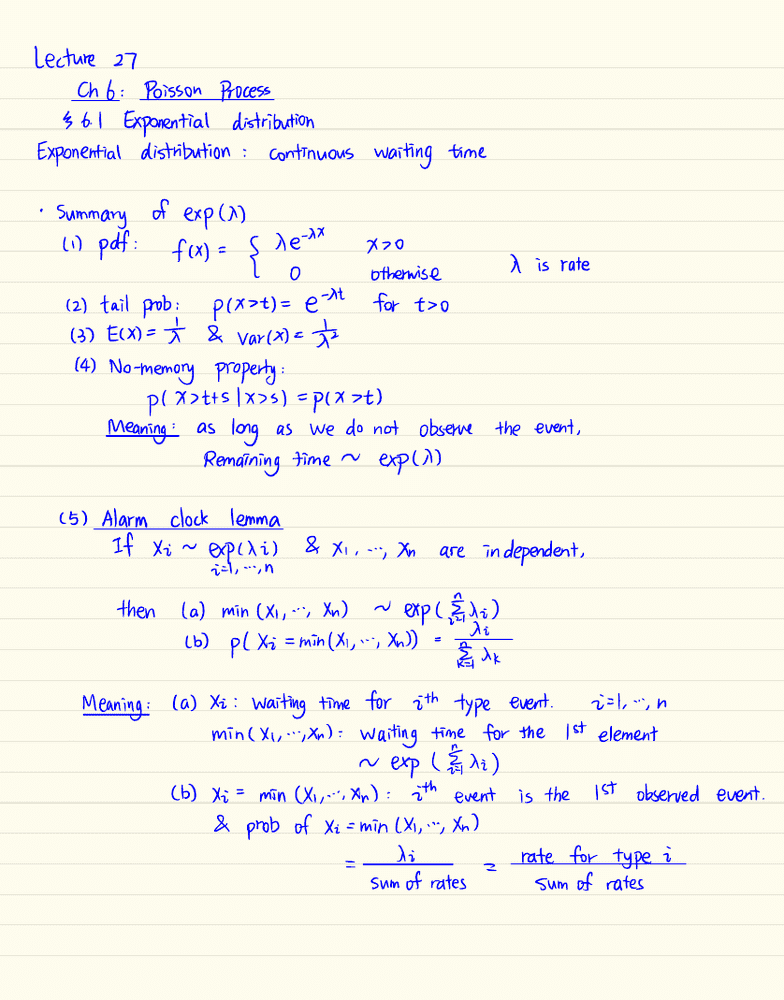# STAT 333 Lecture 27: STAT 333 Lecture 27 Spring 2016

62 views3 pages
School
Department
Course
ProfessorLecture 27
Chb :Poisson Process
36-1 Exponential distribution
Exponential distribution :continuous waiting time
.Summary of exp (D)
*,
li )pdf :fcx )={Re X
0Otherwise Xis rate
(2) tail pnb :p(x>t)= et 't for t>o
(3) ECX )=¥ &Varma at
(4) No -memory property :
pl Xsttslx >s )=p (X>t )
Meaning : as long as We do notobserve the event ,
Remaining time ~exp (D)
(5) Alarm clock lemma
If Xi ~ expcxi )&Xi ,
";Xn are independent ,
isl ,
-.'in
then (a) min (X,
"
'
,Xn )~e*p( ¥,di )
lb )p( Xi =mih( Xi ,
,.
.
,Xn ))=
tXK
Meaning :(a) Xii waiting time for ith type event . it ,
";n
min (Xi ,
..;Xn ):Waiting time for the 1st element
~exp (FE
,xi )
(b) Xi 's min (Xiii ,Xn ): 5th event is the 1st observed event .
&pnob of Xi = min (X,
''iXn )
=
di
=
rate for type i
Sum of rates sum of rates
Unlock document

This preview shows page 1 of the document.
Unlock all 3 pages and 3 million more documents.

Already have an account? Log in

# Get access

Grade+
\$10 USD/m
Billed \$120 USD annually
Homework Help
Class Notes
Textbook Notes
40 Verified Answers
Study Guides
1 Booster Class
Class+
\$8 USD/m
Billed \$96 USD annually
Homework Help
Class Notes
Textbook Notes
30 Verified Answers
Study Guides
1 Booster Class